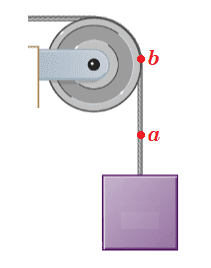# Angular acceleration problem for a pulley used to raise an elevator

• leggythegoose
Please pay attention and try to follow his hints.Also, please quote the post to which you are responding when you post. It makes the conversation easier to follow.In summary, the conversation discusses calculating the linear acceleration of an elevator and the tangential acceleration of a point on a rotating disk. The linear acceleration is found by multiplying 1/8 g (1.22625 m/s^2) by the radius (1.25 m), giving a result in units of m^3/s^2, which is incorrect. To find the tangential acceleration, the correct equation is a = rω^2, where ω is the angular velocity. Omega (ω) is not the same as the linear acceleration (a) and should

#### leggythegoose

Homework Statement
In a charming 19th-century hotel, an old-style elevator is connected to a counterweight by a cable that passes over a rotating disk 2.50 m in diameter. The elevator is raised and lowered by turning the disk, and the cable does not slip on the rim of the disk but turns with it. To start the elevator moving, it must be accelerated at 1/8 g. What must be the angular acceleration of the disk, in rad/s^2?
Relevant Equations
constant angular acceleration equations
I tried to multiply 1/8 g (1.22625) by the radius (1.25 m) and got 1.53 rad/s^2. This is actually the linear acceleration of the elevator. How do I get the angular acceleration of the disk? Thanks!

leggythegoose said:
I tried to multiply 1/8 g (1.22625) by the radius (1.25 m) and got 1.53 rad/s^2. This is actually the linear acceleration of the elevator.
The linear acceleration of the elevator is 1/8 g.

leggythegoose said:
How do I get the angular acceleration of the disk? Thanks!
What is the tangential acceleration of a point on the rim of the rotating disk? How is the tangential acceleration related to the angular acceleration of the disk?

•Lnewqban
TSny said:
The linear acceleration of the elevator is 1/8 g.

What is the tangential acceleration of a point on the rim of the rotating disk? How is the tangential acceleration related to the angular acceleration of the disk?
So I know that r is 1.25 m but how would I find alpha to calculate the tangential acceleration?

leggythegoose said:
So I know that r is 1.25 m but how would I find alpha to calculate the tangential acceleration?
You don't need to know ##\alpha## in order to find the tangential acceleration of a point on the rim of the disk. Hint: "the cable does not slip on the disk"

•Lnewqban
TSny said:
You don't need to know ##\alpha## in order to find the tangential acceleration of a point on the rim of the disk. Hint: "the cable does not slip on the disk"
Do I multiply r and omega? So it would be 1.25x1.91 = 2.39 and 2.39 is the tangential acceleration?

leggythegoose said:
Do I multiply r and omega? So it would be 1.25x1.91 = 2.39 and 2.39 is the tangential acceleration?
Go back to post #4.

http://hyperphysics.phy-astr.gsu.edu/hbase/rotq.html#rq

Then, try again.leggythegoose said:
I tried to multiply 1/8 g (1.22625) by the radius (1.25 m) and got 1.53 rad/s^2
Note that if you multiply 1.22625 m/s2 by 1.25 m you get a result in units of m2/s2, not rad/s2

This is a hint that you should not be multiplying those two quantities.

•Lnewqban
leggythegoose said:
Do I multiply r and omega? So it would be 1.25x1.91 = 2.39 and 2.39 is the tangential acceleration?
No. Note that multiplying the units of ##\omega## by the units of ##r## does not give units for acceleration. [EDIT: A similar units problem was noted by @jbriggs444 ]What is the magnitude of the linear acceleration of point ##a## of the cable? What is the magnitude of the linear (tangential) acceleration of point b on the rim of the disk assuming that the cable does not slip on the disk?

Last edited:
•jbriggs444 and Lnewqban
leggythegoose said:
Do I multiply r and omega? So it would be 1.25x1.91 = 2.39 and 2.39 is the tangential acceleration?
The number 1.91 which you are calling "omega" -- you got that by multiplying the linear acceleration (a = 1/8 g = 1.22625 m/s2) by the radius (r = 1.25 m) and then again by the radius (r = 1.25 m).

Omega (##\omega##) is the greek letter conventionally used to denote angular velocity, usually with units of rad/s.

The calculation you used cannot possibly yield an angular velocity in rad/s. Instead, it yields a result in units of m3/s2.

Then you multiply by 1.25 m yet again, apparently in the hopes that this finally will yield an angular acceleration (in units of m4/s2).

Stop multiplying by ##r## already. You are going the wrong way!

@TSny is trying hard to lead you to a correct calculation.

Last edited:
•Lnewqban and TSny